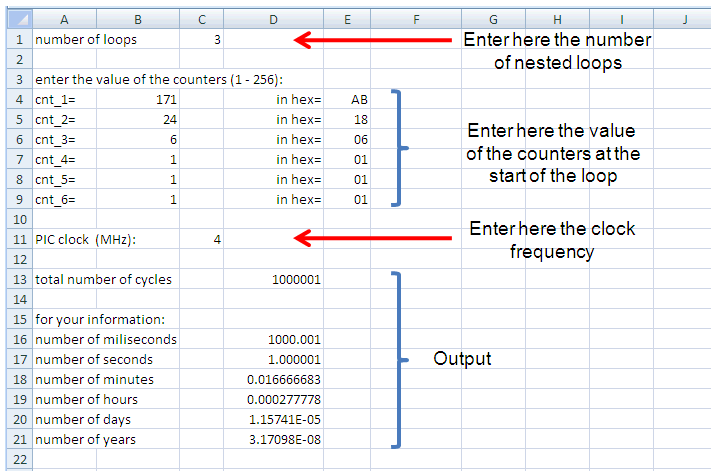# Implementing a Delay in a PIC Program

## Ronald Dekker

Sometimes it can be necessary to implement a fixed delay in a PIC assembly program. As with any processor, this is done by executing just a lot of useless instructions, preferably organized in one or more loops. The PIC instruction set includes a very useful instruction for this: “decfsz” or in English “decrement register so and so, and skip the next instruction if the result after decrementing is zero.” With this instruction it is very simple to make a short delay loop.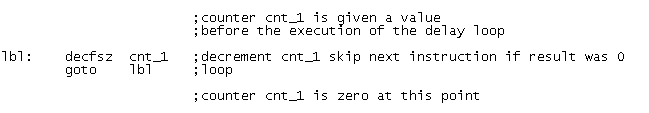The piece of code shown above is the shortest PIC assembly implementation of a delay loop. It consists of only two instructions and uses one register. We assume that the program code which precedes the delay loop has initialized the register to a certain value. The first instruction decrements the register. When the result is not zero, the second instruction is executed. This is a jump to the first instruction so that we have a repetition of the actions just described. At a certain point the register reaches the value zero. When this happens the “decfsz” skips the “goto” statement, and the delay loop is finished.

The first question that we can ask ourselves is how long does it take to excecute this delay loop for an arbitrary starting value of the register. To answer that question it is instructive to assume a certain starting value of the register of say 4, and to re-write the loop as a linear piece of code without the jumps.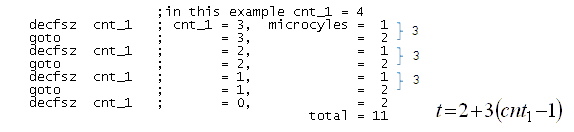From the datasheet of the PIC processor we know that almost all instructions of the processor are executed in one micro-cyle. One micro-cycle takes exactly four clock cycles. At a clock frequency of 4 MHz, one micro-cycle takes 4*250ns = 1us. So at 4 MHz clock most instructions execute in 1us. The only instructions which take 2 micro-cycles are instructions which modify the value of the program counter. An example of such an instruction is the “goto” instruction. The “decfsz” instruction on the other hand can take either one or two instructions. When the outcome of the decrement is not equal to zero, the next instruction is executed and the instruction takes just one micro-cycle. When the result was zero however, the program counter is modified so that the next instruction is skipped, and the instruction takes two micro-cycles.

In the example above both the value of the register, as well as the length of the instructions (in micro-cycles) has been listed. The formula for the delay of the loop is easily found to be t=2+3(cnt-1). No great mysteries here!

At this point it is important to note that the smallest value the register can take is 01H and not 00H! This is because the decrement is performed before the instruction evaluates the value. So if it were 00H, the “decfsz” instruction would first decrement 00H to FFH. In fact 00H is not the smallest, but the largest value the register can hold. The value range for a register is therefore 01H,...,FFH,00H; corresponding to decimal values 1,...,255,256. The longest delay which can be generated with a single loop thus amounts to 2+3(256-1)=2+3*255=767 micro-cycles, or with a clock frequency of 4 MHz: 767us, still less than 1ms. If a longer delay is needed, a second delay loop can be implemented over the first one.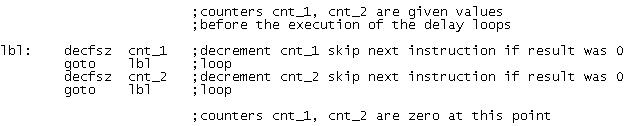A double or nested loop delay counter is listed in the program example shown above. Compared to the single loop counter two instructions are added which implement a second loop, over the already existing first one. After start of the program first cnt_1 is counted down by the first “decfsz” and “goto” instructions. When finally cnt_1 reaches zero, cnt_2 is decremented, and the inner loop is executed again. It is important to note that when the inner loop is executed for the first time, the inner loop is executed (cnt_1-1) times. However, after the first execution of the inner loop, cnt_1 equals 00H. So every next time the inner loop is executed (256-1)=255 times. If this does not generate enough delay, another loop can be added over the existing two. Obviously this can be expanded to n-loops.

This raises the question how can we calculate the delay of n nested loops counting down n registers which are filled with arbitrary values at the beginning of the loop? Before we answer that question, we will first look at the special case when we have n nested loops, but with the n registers all initialized (cleared) to the maximum value of 00H (256).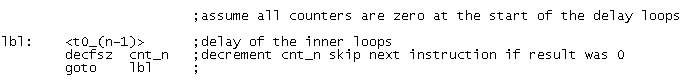In the piece of symbolic code above, an n-th loop is added over an already existing delay counter with n-1 loops. Only the instructions for the new loop are shown. The delay of the n-1 inner loops is represented by t0_(n-1). The 0 in t0(n-1) indicates that all the registers were set to zero at the beginning of the execution of the loop. As with the preceding examples we remove the loop and write the program as a linear set of instructions: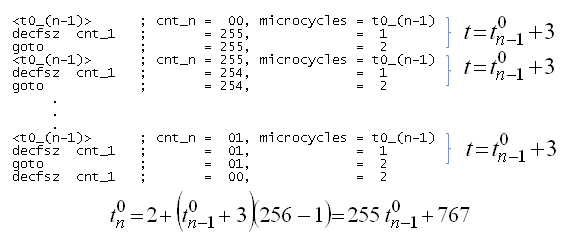The recursive formula derived above gives the delay of the n-th added loop. This formula contains an unknown: t0_(n-1). To find this unknown, we have to fill in again the same formula, this is why we say it is recursive. As an example we show the calculation of the delay of 3 nested loops: t0_3. To calculate t0_3 we need t0_2, for which we need t0_1 for which we need t0_0. But since t0_0=0 we can now calculate t0-1, t0_2 and finally t0_3: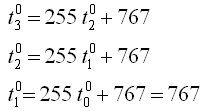Using this recursive formula it is possible to calculate the delay for any arbitrary number of n nested loops with 00H as starting value for the n counters. Here is for n=1 to 10 the length of such a loop in micro-cycles. Remember that for an oscillator frequency of 4 MHz one micro-cycle corresponds to 1 us.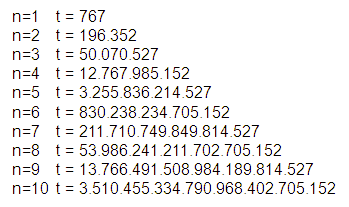We now turn to the slightly more complicated job of calculating the delay of n nested loops with arbitrary starting values of the n loop counters. What is different in this case is, as explained, that the first time the inner loop is calculated the initial value of the counter of the inner loop is in general unequal to 00H (or 256 dec). We denote the delay of this loop with t1_(n-1), the 1 indicating that the counter was not equal to 00H. The second time the inner loop is executed however, the value of the counter is 00H, so we denote the delay with t0_(n-1).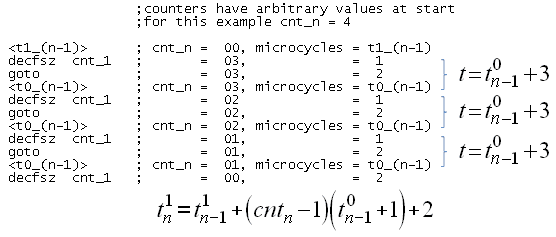In the example above we have, just as in the previous case when all the counters were cleared, linearized a piece of code in which the outer loop counter was set to 4. As mentioned, the inner loop execution time is written as t1_(n-1). The next time the inner loop is executed, all the inner loop counters are cleared so that their execution time is written as t0_(n-1). With these definitions we come to the recursive formula given above.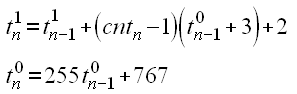We now have two recursive formulas who together yield the length of any number of nested loops with arbitrary set loop counters. The formulas were easily implemented in excel. The screen dump below shows the upper part of the spread sheet. A maximum of 6 nested loops are implemented. The number of loops is set in the first line. Next the starting values for the counters can be entered. The program returns the appropriate hex value for convenience and checks if the values are within range. Note that the values for the counters are entered in decimal format with a value ranging from 1-256. As explained te decimal value of 256 corresponds to 00 in hex format. Only the counters for the specified number of nexted loops are relevant. The final thing that can be set is the processor clock frequency. The spreadsheet calculates the number of micro-cycles the total delay loop takes and then calculates the corresponding execution time.